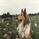# Vervoort Volatility Bands [LazyBear]

3263 lượt xem
3263
This is Mr. Vervoort's take on volatility bands. Sticking to his style, he uses highly smoothed data everywhere, also improves on the way the bands are calculated. Is this better than others? I will let you guys decide :)

List of my other indicators:
```//
// @author LazyBear
// List of all my indicators:
//
study("Vervoort Volatility Bands [LazyBear]", shorttitle="VVB_LB", overlay=true)
src = hlc3
al = input(8, title="Average Length")
vl = input(13, title="Volatility Length")
df = input(3.55, "Deviation Multiplier")
lba = input(0.9, "Lower Band Adjustment Multiplier")

typical = src >= src ? src - low : src - low
deviation = df * sma(typical, vl)
devHigh = ema(deviation, al)
devLow = lba * devHigh
medianAvg = ema(src, al)

MidLine = plot (sma(medianAvg, al), color=gray, title="MidLine")
UpperBand = plot (ema(medianAvg, al) + devHigh, color=red, linewidth=2, title="UpperBand")
LowerBand = plot (ema(medianAvg, al) - devLow, color=green, linewidth=2, title="LowerBand")```
List of my free indicators: http://bit.ly/1LQaPK8
List of my indicators at Appstore: http://blog.tradingview.com/?p=970grahvity
You forgot KC, STARC and my COG channel :P
Phản hồiLazyBear
I like the DEnvelope. Where can I find the formula for it? Thanks
Phản hồioaksacorn
It is in my published list